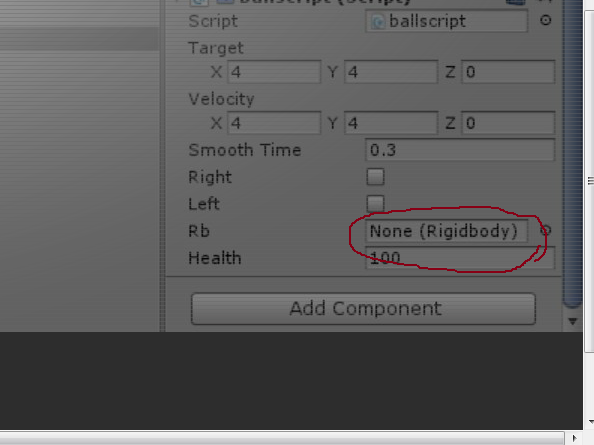# rigidbody not moving

Hey guys, i’m new to unity but I have android studio experience looking to see how the game industry is !

So i have a ball which is a rigidbody with a circular collider which is located between two walls. The ball is moved to the right when right arrow is pressed and left when left arrow is pressed with transform.position . The initial problem was that the ball was going through the walls even though it shouldn’t, because I was using transform.position instead of rigidbody.moveposition which was messing with the physics engine (from what I read here). Now I am trying to use rigidbody.moveposition but the object is not moving anymore :

Here’s a video to help you guys get my situation, everytime you hear a fairly loud bang it means i’m hitting either left or right mouse on keyboard :

http://screencast.com/t/7SYxQXfCwMeZ

(Script attached to the ball)

``````using UnityEngine;
using System.Collections;

public class ballscript : MonoBehaviour {

public Vector3 target = Vector3.zero;
public Vector3 velocity = Vector3.zero;
public float smoothTime = 0.3F;
public bool right = false;
public bool left = false;
public Rigidbody rb;

public float health = 100;

// Use this for initialization
void Start ()
{
rb = GetComponent<Rigidbody> ();
}

IEnumerator Example() {
yield return new WaitForSeconds(0.0525f);
left = false;
right = false;
}

// Update is called once per frame
void FixedUpdate ()
{

if (right == true) // OBJECT DOESN'T MOVE.
{

target.x = rb.position.x + 18;
target.y = rb.position.y;
target.z = -1;
rb.MovePosition(Vector3.SmoothDamp (transform.position, target, ref velocity, smoothTime));

StartCoroutine(Example()); // NEVER CALLED.

}

if (left == true) // OBJECT DOES MOVE.
{

target.x = transform.position.x - 18;
target.y = transform.position.y;
target.z = -1;
transform.position = Vector3.SmoothDamp (transform.position, target, ref velocity, smoothTime);
StartCoroutine (Example ()); //CALLED.

}

}

void OnBecameInvisible()
{
Time.timeScale = 0;
Destroy (gameObject);
Debug.Log ("BALL HAS BEEN DESTROYED");
}

void Update()
{

if (Input.GetKeyDown (KeyCode.RightArrow))
{

right = true;
}

if (Input.GetKeyDown (KeyCode.LeftArrow))
{
left = true;
}

}
}
``````

I hope someone can help me, i’m really determined to fix this and continue on my endeavor.